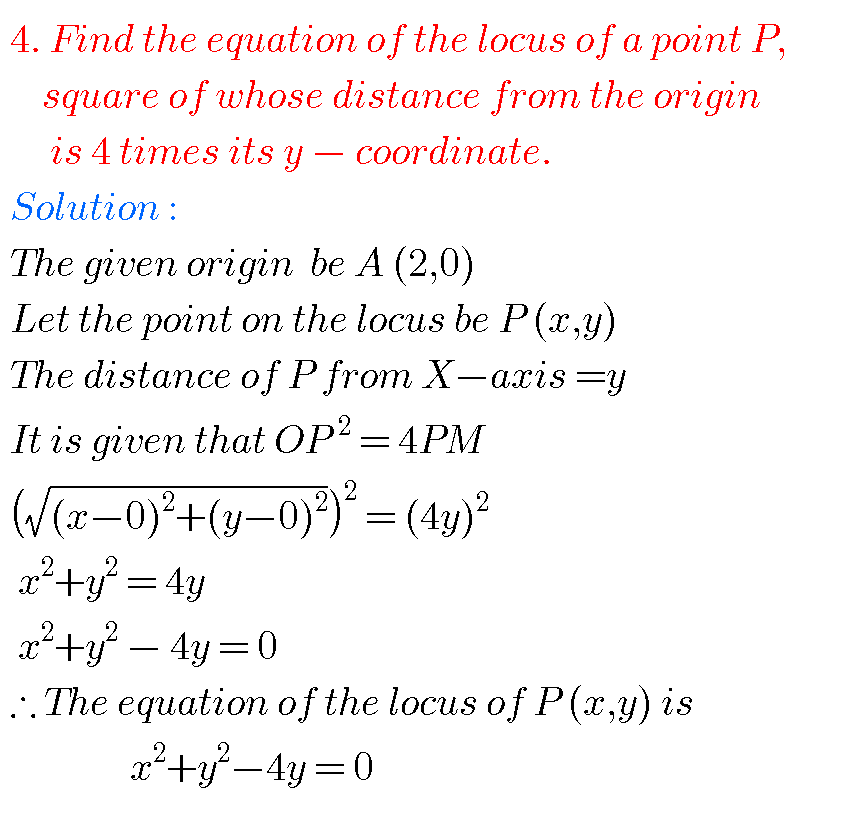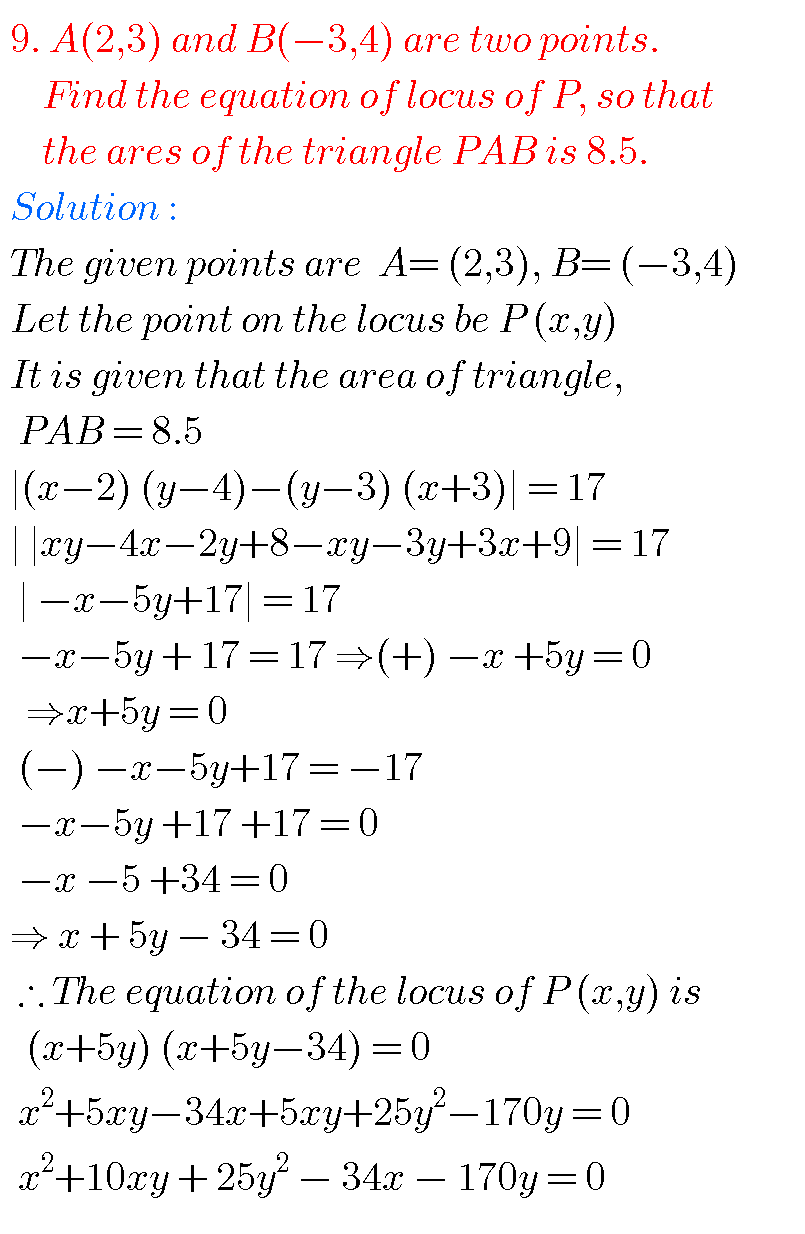# LOCUS, INTER FIRST YEAR MATHEMATICS 1B CHAPTER 1 PROBLEMS WITH SOLUTIONS

Mathematics Intermediate1b solutions for some problems are available here. These are very simple to understand.

Study the textbook lesson very well.

Practice the example problems and solutions given in the textbook.

Observe the given solutions and try them in your own method.

Textbook solutions for Locus

Exercise 1(a)

You can also see

Inter Maths 1A textbook solutions

Inter Maths 1B textbook solutions

Inter Maths IIA textbook solutions

Inter Maths IIB textbook solutions

M

# LOCUS SOLUTIONS 1B INTER Coordinate GeometryProblem 2## LOCUS, INTERMEDIATE FIRST YEAR MATHEMATICS 1B CHAPTER 1 PROBLEMS WITH SOLUTIONS### LOCUS, INTERMEDIATE FIRST YEAR MATHEMATICS 1B CHAPTER 1 PROBLEMS WITH SOLUTIONS,COORDINATE GEOMETRY LOCUS INTER 1B

Some moreYou can also see the solutions of junior inter maths 1B

1Locus

Straight lines sa

Straight lines la

12. Rate measure

Maths real numbers solutions class 10

Note : Observe the solutions and try them in your own methods.

### 6 thoughts on “Locus,Intermediate first year Mathematics 1B chapter 1 problems with solutions,Junior inter Coordinate Geometry”

1.Congratulations for this nice platform.
Mathematics is life.

2.Thanks for sharing this knowledge

3.Very happy,it helped me a lot for the preparation.

4.Good very happy to your explanation process

5.Good